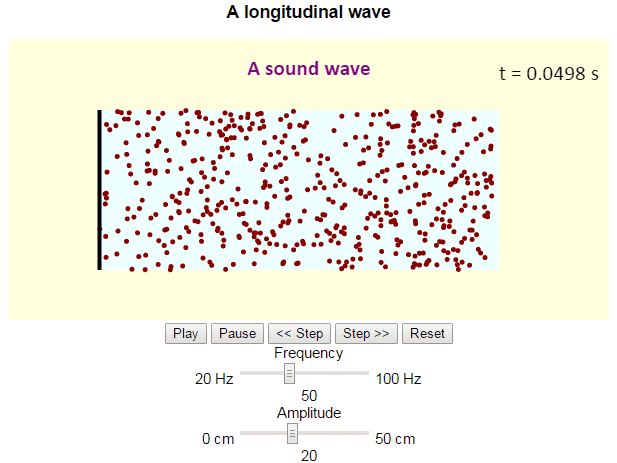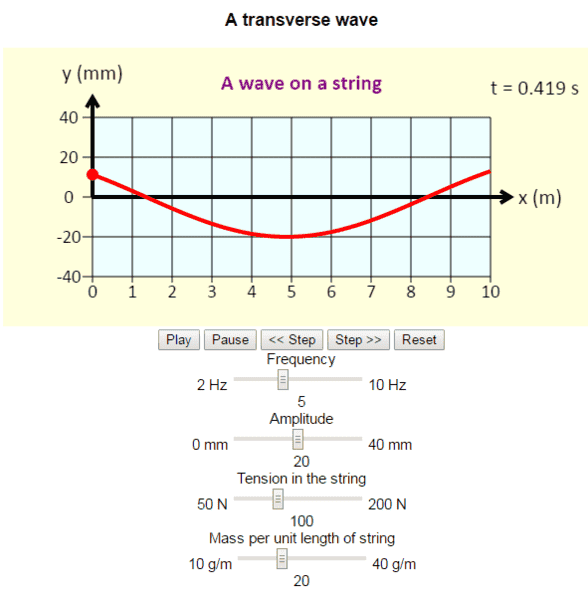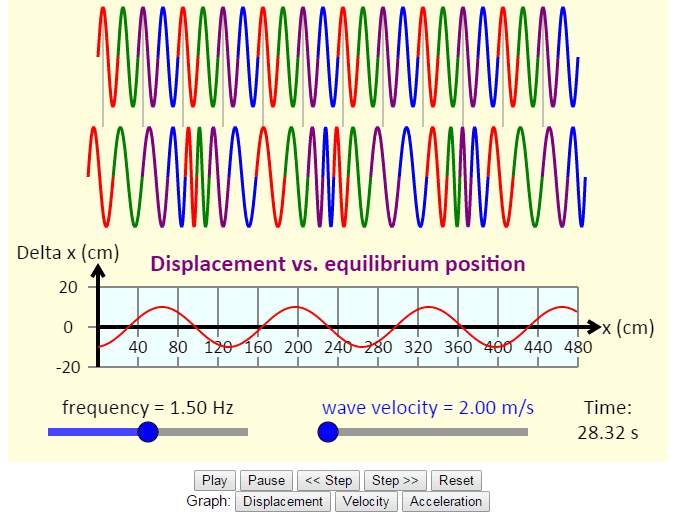# Why longitudinal waves are waves?

i know it's an absurd question, but why are longitudinal waves called waves although they aren't wave-like?

Drakkith
Staff Emeritus
i know it's an absurd question, but why are longitudinal waves called waves although they aren't wave-like?

They are wave-like. They behave according to the wave equation.•davenn and russ_watters
Dale
Mentor
2021 Award
i know it's an absurd question, but why are longitudinal waves called waves although they aren't wave-like?
They diffract, refract, and reflect. I am not sure what is left to be considered wave-like

russ_watters
Mentor
Perhaps you mean they don't look instantly recognizable as sine waves? There's a sine wave in there, it's just rotated 90 degrees, flattening it.

jbriggs444
Homework Helper
Perhaps you mean they don't look instantly recognizable as sine waves? There's a sine wave in there, it's just rotated 90 degrees, flattening it.
If you plot pressure (or whatever) versus position and look at the resulting graph as it evolves over time, the "90 degrees" is not physical. It is the fact that there are two [mostly] orthogonal variables that are plotted at 90 degrees from one another on the graph paper.

russ_watters
Mentor
If you plot pressure (or whatever) versus position and look at the resulting graph as it evolves over time, the "90 degrees" is not physical. It is the fact that there are two [mostly] orthogonal variables that are plotted at 90 degrees from one another on the graph paper.
I think we mostly agree, but will separate:

The part that is physical is the amplitude - the motion of the particles - is in the x-direction for a longitudinal wave. Since that is the same axis as the propagation of the wave, you can't see it (a graphic just looks like a bunch of fuzzy vertical stripes). If you plot it in the y axis - rotate it 90 degrees - it becomes visible, but is just a representation of what actually happens.

What isn't physical is the various properties you can choose to plot - pressure or displacement vs distance or time. I think this is what you were referring to.

Contrast that with a transverse wave, which literally plots itself as a sine wave. You could literally draw an x-y axis on the wall behind a shaken rope and see the sine wave.

•jbriggs444

sophiecentaur
Gold Member
i know it's an absurd question, but why are longitudinal waves called waves although they aren't wave-like?
Not too absurd, actually - if you are comparing them with waves on water, where the motion of the water appears to be 'up and down'. However, as the water moves 'obviously' up and down, it is also moving forward and backwards (taking water from the troughs and putting it in the peaks). i.e. there is also a significant Longitudinal component. Most cases are a mixture of transverse and longitudinal waves, once the amplitude gets large. Even Electromagnetic Waves passing through a medium can have slight longitudinal component, despite what we say about radio waves being Transverse.
You just can't win.

olivermsun
They are wave-like. They behave according to the wave equation.Is that a strict requirement?Drakkith
Staff Emeritus
Is that a strict requirement?I thought so. Am I mistaken?

robphy
Homework Helper
Gold Member
http://physics.bu.edu/~duffy/HTML5/longitudinalwave.htmlhttp://physics.bu.edu/~duffy/HTML5/wave.htmlhttp://physics.bu.edu/~duffy/HTML5/longitudinal_wave.html#### Attachments

olivermsun
I thought so. Am I mistaken?
There are many examples of waves that do not behave much like the wave equation, so I don't think the wave equation can be the defining feature for what makes a wave.

I am also not sure I agree with Dale's answer that longitudinal waves "diffract, refract, and reflect," so what else could there be for them to be considered waves? A longitudinal wave in a slinky doesn't really have to diffract or even refract to be recognizable as a wave. The slinky wave does reflect, but then again so does a billiard ball rolling inside the slinky (assuming the ends are closed), and that clearly isn't a wave. It seems to me that there has to be more to being a wave than properties that follow from, e.g., the wave equation.

Drakkith
Staff Emeritus
There are many examples of waves that do not behave much like the wave equation, so I don't think the wave equation can be the defining feature for what makes a wave.

Can you give me an example?

olivermsun
Ocean waves are one familiar example.

Drakkith
Staff Emeritus
Ocean waves are one familiar example.

How do those not obey the wave equation?

•Delta2
Delta2
Homework Helper
Gold Member
Ocean waves are one familiar example.
Aren't ocean waves a mix of longitudinal and transverse waves, which each of them obeying the wave equation?
Is it because we have dispersion in ocean waves? Even with dispersion, each unique frequency component obeys a unique wave equation, but yes the wave as a whole doesn't obey the standard wave equation.

What is the definition of wave for you?

Last edited:
olivermsun
How do those not obey the wave equation?
I'm not sure what kind of answer you're looking for here. For starters, water waves obey a different (Laplace's) equation.

olivermsun
Aren't ocean waves a mix of longitudinal and transverse waves, which each of them obeying the wave equation?
No. The motion of ocean waves is orbital. The water moves in both the longitudinal and transverse directions, but it isn't a mix of two distinct waves. In fact, the two types of motion can't be independent if you want to satisfy conservation of mass.

Is it because we have dispersion in ocean waves? Even with dispersion, each unique frequency component obeys a unique wave equation, but yes the wave as a whole doesn't obey the standard wave equation.
It might be more useful to say that we have dispersion in ocean waves because they are governed by a different physics than the wave in a string.

What is the definition of wave for you?
It's pretty hard to come up with a definition of a wave that encompasses all the things we think of as waves. "You'll know it when you see it" might be about as good as it gets. To quote Whitham (1974):
Various restrictive definitions can be given, but to cover the whole range of wave phenomena it seems preferable to be guided by the intuitive view that the wave is any recognizable signal that is transferred from one part of the medium to another with a recognizable velocity of propagation...This may seem a little vague, but it turns out to be perfectly adequate and any attempt to be more precise appears to be too restrictive; different features are important in different types of waves.

•nasu
Delta2
Homework Helper
Gold Member
@olivermsun what is the equation that governs ocean waves? I suspect the equation or set of equations isn't linear and that's one of the reason we cant speak about separate longitudinal and transverse components? You say that they satisfy Laplace's equation, but Laplace's equation is
1) linear
2) doesn't contain the time variable, so the full equation must be something else.

sophiecentaur
Gold Member
Is that a strict requirement?The equation resulting from solving an equation of the 'motion' of the particles (or variation of the fields) for all waves has so much in common that I would say it is a better description than anything else about a general Wave.
The sine wave thing is really a bit of a red herring here because the shape of a wave depends more on the variations of the generator that causes the waves. There are many waves of very short duration (single pulses are very common) that are definitely not sinusoidal yet they are still waves.

olivermsun
@olivermsun what is the equation that governs ocean waves?
As I said above, it’s Laplace’s equation (elliptic), not the wave equation (hyperbolic). This is true not just for water waves but for a whole class of important flows.

I suspect the equation or set of equations isn't linear and that's one of the reason we cant speak about separate longitudinal and transverse components?
The physical interpretation for why the longitudinal and transverse components of a water wave have to be related is that the water volume has to be conserved. For example, if the surface moves upward, then water has to move in from the sides and/or below to fill that volume. This remains true for linear(ized) waves.

You say that they satisfy Laplace's equation, but Laplace's equation is
1) linear
2) doesn't contain the time variable, so the full equation must be something else.
1) see above
2) First of all, there are boundary conditions, i.e., no flow through the free surface (this usually gets linearized) and the bottom boundary. Secondly, if you have Laplace’s equation in terms of pressure or velocity (potential), then that’s where you have the time derivatives.

olivermsun
The equation resulting from solving an equation of the 'motion' of the particles (or variation of the fields) for all waves has so much in common that I would say it is a better description than anything else about a general Wave.
But it is generally untrue that wave solutions look alike, except that they propagate while remaining “recognizable” in some way.

The sine wave thing is really a bit of a red herring here because the shape of a wave depends more on the variations of the generator that causes the waves. There are many waves of very short duration (single pulses are very common) that are definitely not sinusoidal yet they are still waves.
I agree that the “sine wave criterion” is a red herring, but you also seem to mixing up several different phenomena.

A hyperbolic wave, like the linear wave in the string, can travel very long distances while retaining almost any initial shape, including isolated pulses.

On the other hand, the shape of dispersive waves can evolve to be nearly independent of the details of their generation. Think of nearly monochromatic (sinusoids) “sets” of ocean waves arriving at a surfers’ beach.

If nonlinearity is added to the system, then you can have yet another possibility: special solutions (e.g., solitons) can arise in which nonlinearity and dispersion balance each other out. In this case certain waves propagate without changing shape, while most other waves still look dispersive.

•nasu
sophiecentaur
Gold Member
But it is generally untrue that wave solutions look alike, except that they propagate while remaining “recognizable” in some way.

I agree that the “sine wave criterion” is a red herring, but you also seem to mixing up several different phenomena.

A hyperbolic wave, like the linear wave in the string, can travel very long distances while retaining almost any initial shape, including isolated pulses.

On the other hand, the shape of dispersive waves can evolve to be nearly independent of the details of their generation. Think of nearly monochromatic (sinusoids) “sets” of ocean waves arriving at a surfers’ beach.

If nonlinearity is added to the system, then you can have yet another possibility: special solutions (e.g., solitons) can arise in which nonlinearity and dispersion balance each other out. In this case certain waves propagate without changing shape, while most other waves still look dispersive.
This is a B level thread. It's easy to leave an OP behind if a thread gets too advanced.
I guess the easiest catch-all description of waves is that they transfer Energy by variations in one or two variables in time and distance without any net flow of material. But I'm sure someone will quote a 'wave' that doesn't follow that description 100%.

•Delta2
sophiecentaur